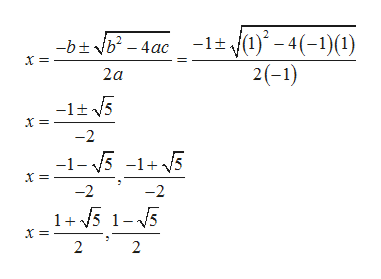Question
1 views

How do I solve this equation? And how do I determine what type of solution it is?

check_circle

Step 1

Given equation is

Step 2

By comparing the given equation by ax^2 +bx+c=0, we have a=-1, b=1 and c=1.Thus, the roots of given equation is given byhelp_outlineImage Transcriptionclose-1+y(1)* - 4(-1)(1) 2(-1) btb- 4ac x 2a -lt 5 -2 -1- 5 -15 -2 -2 1+5 1-5 2 2 fullscreen
Step 3

Since discriminant =b^2-4ac>0, so the roots of ...

### Want to see the full answer?

See Solution

#### Want to see this answer and more?

Solutions are written by subject experts who are available 24/7. Questions are typically answered within 1 hour.*

See Solution
*Response times may vary by subject and question.
Tagged in

### Algebra